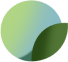# 1 Pair- Standard Classic, or Day Bra Underwire Premium Quality Metal, Thermoplastic Coated- Sizes 30 to 50

87 reviews
Price
\$2.99
Taxes and shipping calculated at checkout

#### Product Information

1 Pair- Sizes 30 to 50, Standard Classic, or Day Bra Underwire Premium Quality Metal, Thermoplastic Coated, for Bra, Lingerie or Swimwear

Sizes: 30, 32, 34, 36, 38, 40, 42, 44, 46, 48, 50 - See below for US cup size reference and link for downloadable PDF size/shape reference. Photos shown on a 1 Inch X 1 inch Grid Mat.

Ideal for day bra, classic bra, and standard shape. For bras, lingerie or swimwear. These underwires are strong and are thermoplastic covered to prevent rust, and for comfort.

Sister or Cross Bra Sizes for reference:
Size 30: 28C = 30B = 32A = 34AA
Size 32: 28D = 30C = 32B = 34A = 36AA
Size 34: 28DD = 30D = 32C = 34B = 36A = 38AA
Size 36: 30DD = 32D = 34C = 36B = 38A = 40AA
Size 38: 30DDD = 32DD = 34D= 36C =38B = 40A = 42AA
Size 40: 32DDD = 34DD = 36D=38C=40B=42A
Size 42: 32E = 34DDD = 36DD = 38D = 40C = 42B = 44A
Size 44: 32F = 34E = 36DDD = 38DD = 40D = 42C = 44B
Size 46: 34F = 36E = 38DDD = 40DD = 42D = 44C = 46B
Size 48: 36F = 38E = 40DDD = 42DD = 44D = 46C = 48B = 50A
Size 50: 34H = 36G = 38F = 40E = 42DDD = 44DD = 46D = 48C = 50B

For printable Standard or Day Bra Underwire Size Reference download the following PDF files:

Sizes 30-42 Measurement Reference Guide: https://bit.ly/2JIxAKp

Sizes 44-50 Measurement Reference Guide: https://bit.ly/2FQgLsS

Material:
AISI 1070 Carbon Steel
PET covered, Thermoplastic tip.
Thickness: 2.76 mm X 0.93 mm

SKU: UndrWireRF325-white-30